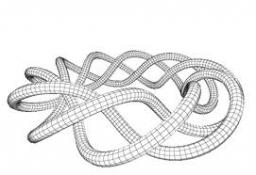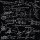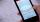# Product

The product of two consecutive odd numbers is 8463. What are this numbers?

Correct result:

x =  91
y =  93

#### Solution:

$y=x+2=93$We would be pleased if you find an error in the word problem, spelling mistakes, or inaccuracies and send it to us. Thank you!Tips to related online calculators

## Next similar math problems:

• DiscriminantDetermine the discriminant of the equation: ?
• ChordsHow many 4-tones chords (chord = at the same time sounding different tones) is possible to play within 7 tones?
• EquationEquation ? has one root x1 = 8. Determine the coefficient b and the second root x2.
• Three unknownsSolve the system of linear equations with three unknowns: A + B + C = 14 B - A - C = 4 2A - B + C = 0
• Elimination methodSolve system of linear equations by elimination method: 5/2x + 3/5y= 4/15 1/2x + 2/5y= 2/15
• CalculationHow much is sum of square root of six and the square root of 225?
• Variations 4/2Determine the number of items when the count of variations of fourth class without repeating is 600 times larger than the count of variations of second class without repetition.Find the roots of the quadratic equation: 3x2-4x + (-4) = 0.
• RootsDetermine the quadratic equation absolute coefficient q, that the equation has a real double root and the root x calculate: ?
• PowerNumber ?. Find the value of x.
• Expression with powersIf x-1/x=5, find the value of x4+1/x4
• Find the 20Find the product and the sum of the roots of x2 + 3x - 9 = 0Solve quadratic equation: 2x2-58x+396=0X+y=5, find xy (find the product of x and y if x+y = 5)In the classroom, students always give candy to their classmates on their birthdays. The birthday person always gives each one candy, and he does not give himself. A total of 650 candies were distributed in the class per year. How many students are in theThe product of a number plus that number and its inverse is two and one-half. What is the inverse of this numberSubstitute the numbers/0,1,2,3/into the equation as x: (x - 1) (x - 3) (x + 1) = 0 Which of them is its solution? Is there another number that solves this equation?